|

# 2017广州建博会,这些新品首秀惊艳你的眼睛

7月8日，第十九届中国(广州)国际建筑装饰博览会在广州中国进出口商品交易会展馆盛大开幕，智能家居、全屋定制、系统门窗、木门/整木家装、五金、厨卫等十余种细分题材的2500多个参展企业，纷纷围绕智能、定制、材料、设计四大主题，进行新产品、新设计和新举措等的“首秀"，诸多新品的采用新技术、新工艺，堪称惊艳，装修情报的编辑第一时间在现场为大家带来了这些不容错过的惊艳产品，赶紧一起来看看吧！

01

智能时代全面来临

巴迪斯吊顶：智能化让使用更简便智能时代，就是吊顶也智能。这是针对吊顶的新品智能开关， 相当于遥控，以前吊顶的每一种功能都要接两条线，非常复杂，现在改成电池，操作方便，即便非专业人士，老人小孩都可以轻松操作。而且配置多个按钮，可以调节冷暖风，广泛用于客厅、卫生间，摆叶可以摆动，还可以换气，干燥功能，吹出热风，抽走湿的空气，这是巴迪斯的专利产品。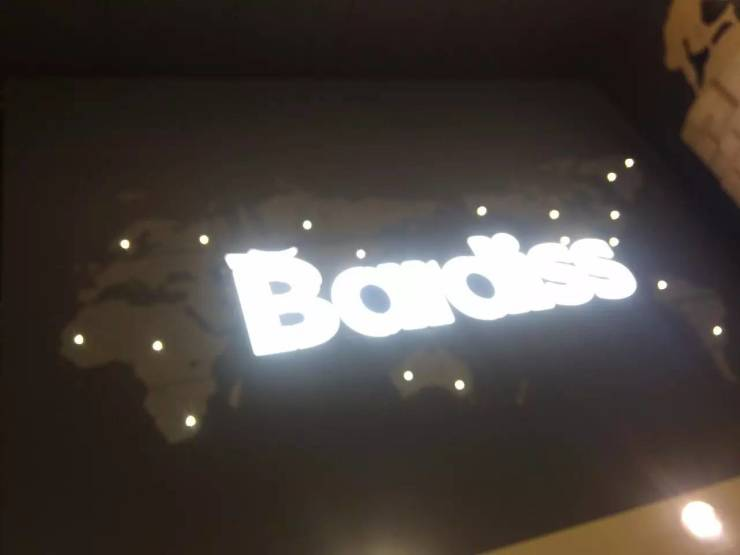今年还有一个最大的特色就是铝制吊顶可以做出各种造型，比如这款吊顶就设计成世界地图，全部是数控做出来的图案，最大可以做到单片板长4米，宽1.8米，这对设备要求非常高，在广东省是领先产品。

贝克洛门窗：智能化成最大亮点

广东贝克洛门窗系统有限公司今年展会最大的亮点便是系列智能产品和通体铝门窗设计，门窗的隔音、隔温和智能化人性化的设计非常惊艳。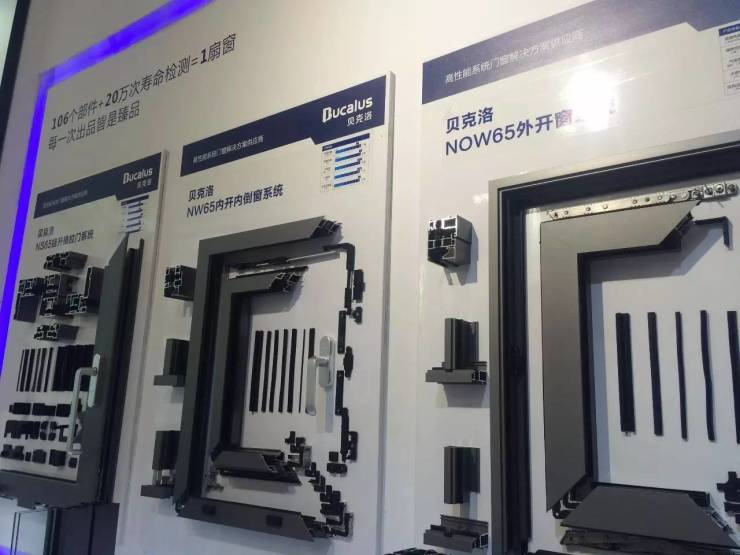全部包括五金都是自己研发的，产品都经过不断地检测，可以自成一个完整的系统，对比其他传统的，外观和材质，注重细节，考虑的更加充分，造型、弧度流畅，手感更好，更加人性化。玻璃是与国内知名供应商如汉斯等合作的产品。五金都是铝合金，表面经过了氧化处理，外观会更加鲜亮，相比传统喷涂会更加耐磨。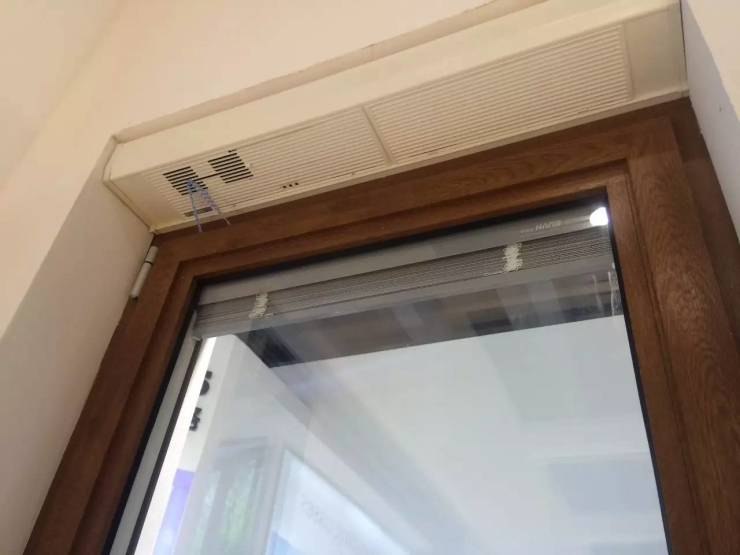窗户增加了通风器，适用于常常出差不在家的消费者，回来之后，天气不好，没办法开窗通风的时候，可以打开通风器，通风器可调节速度，通风换气效果特别好。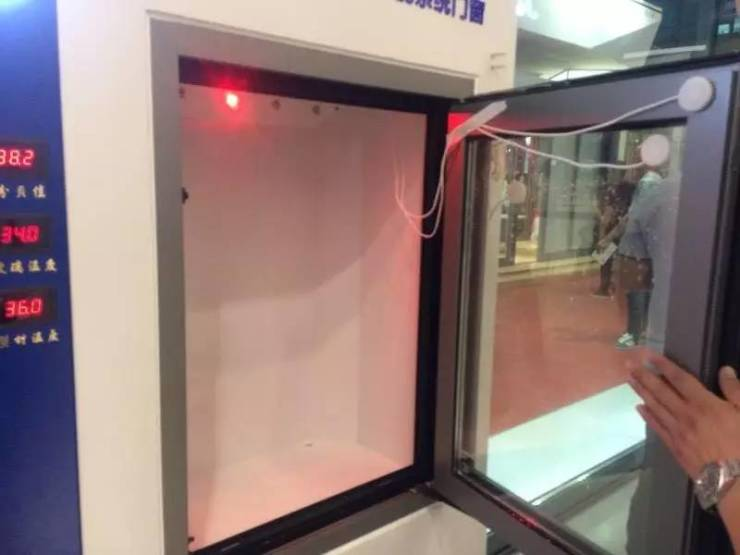这是工作人员现场演示，这款门窗隔温、隔雨、隔音效果非常好，关上之后，室内温度可比室内低十几度，声音低几十分贝。因为门窗做了密封桥设计，不用打胶，同时增加了排水系统，有效隔雨，采用双层中空玻璃，很好地隔音。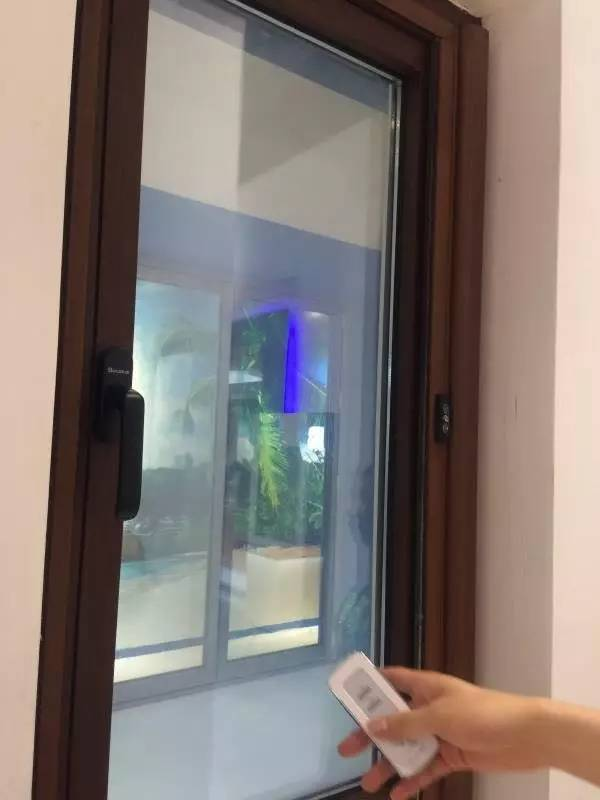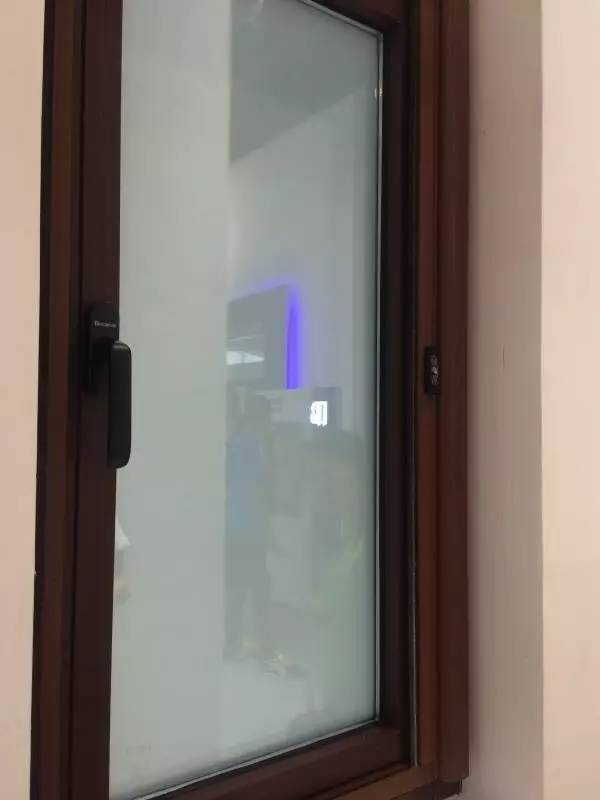这款门窗可电控调节增加玻璃云雾，是新的智能技术，变压系统隐藏在门框处。点击遥控器，玻璃就增加云雾，变得不透明，用于洗浴间非常方便。还可用于一些高端别墅，比如外面是花园，平时可以设置成透明玻璃，视野很好，当主人不在家的时候，可以调节成云雾色不透明，很好地保护隐私。

02

产品更加定制化，满足多样需求

木里木外：更加人性化、细节化

上海木里发展实业有限公司，在本次展会上推出的几款门，设计仍然以现代简约为主，但更加注重人性化，更加贴近消费者的需求，而且设计上更具品质和时尚。材质上，仍然继续主打金丝楠木，在色彩上，本次展会推出了五色系列，特别的是，在黑的系列加入了黛色，新加入了浑水漆，更符合客户要求多样化。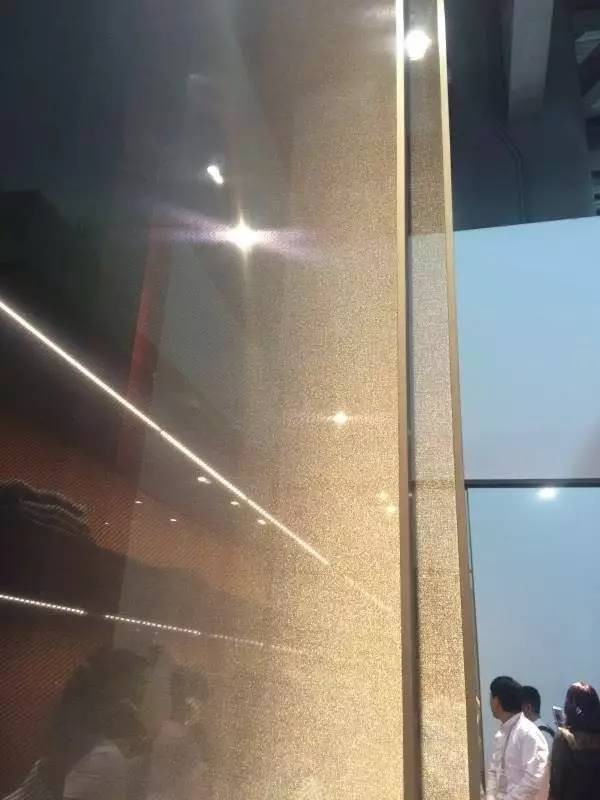新衣帽间系统，全部是夹丝玻璃，设计师找了50多款夹丝，几乎找遍全国和世界各地的夹丝玻璃，才找到这一款唯一适合品牌调性的。铜的结构也是自己研发的。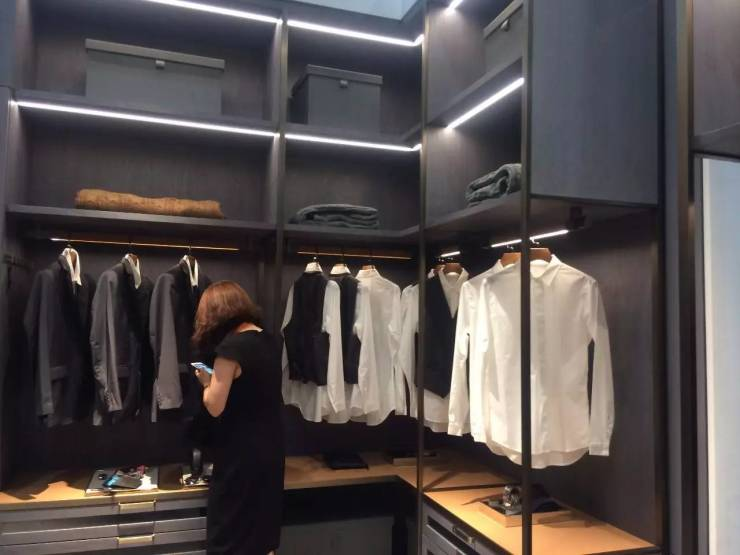男衣帽间，偏灰色，今年用金属支架用来代替原来的木框，更灵活。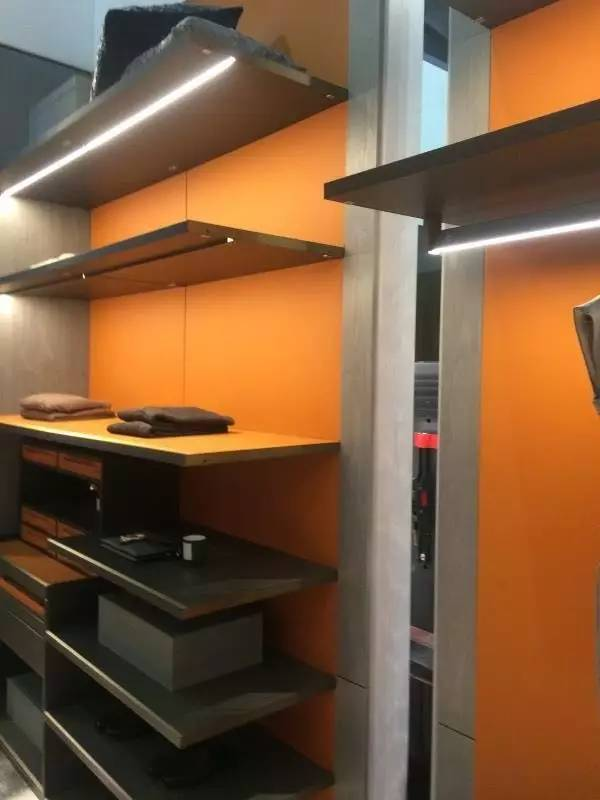新的衣帽间系统，之前的比较传统，今年增加了层板，收纳更丰富，之前是柜体简单框起来，更开放的理念，也体现了木里木外的国际化视野。爱马仕橙的运用，与高端品牌接轨。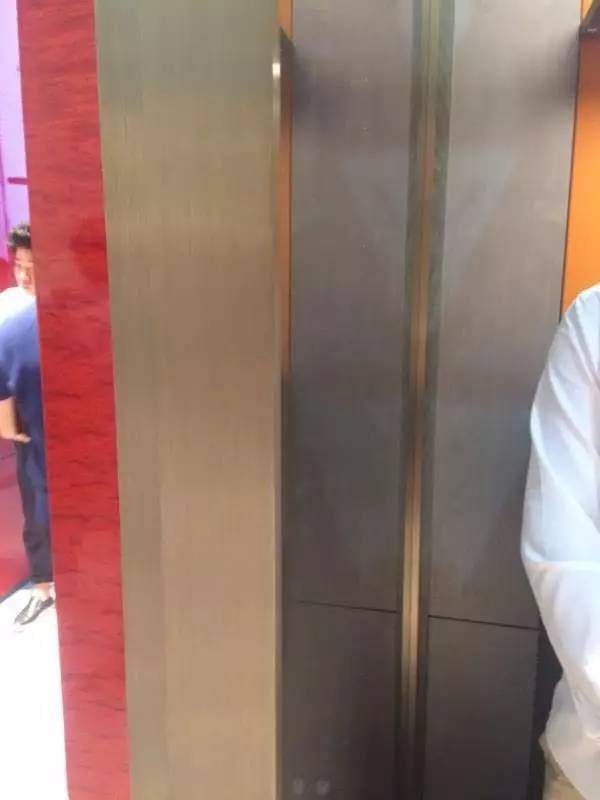门是金属、皮革木皮结合，非常高端，皮革更贴近皮肤温度，更贴近人的理念。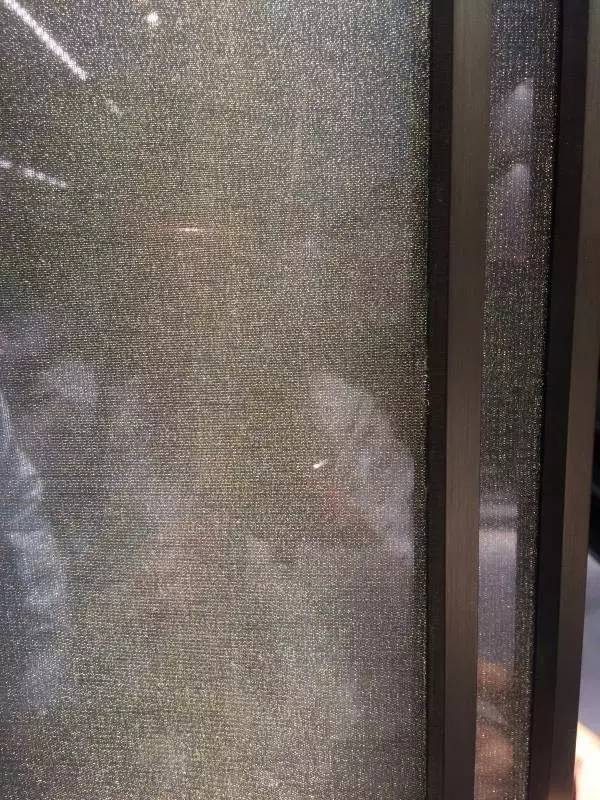推拉门也是皮革与金属结合。他们今年推出了7款门，都非常高，大概3米，全部采用各种拼花，不再是传统的直拼，完全可以由自己设计，进行高端私人订制。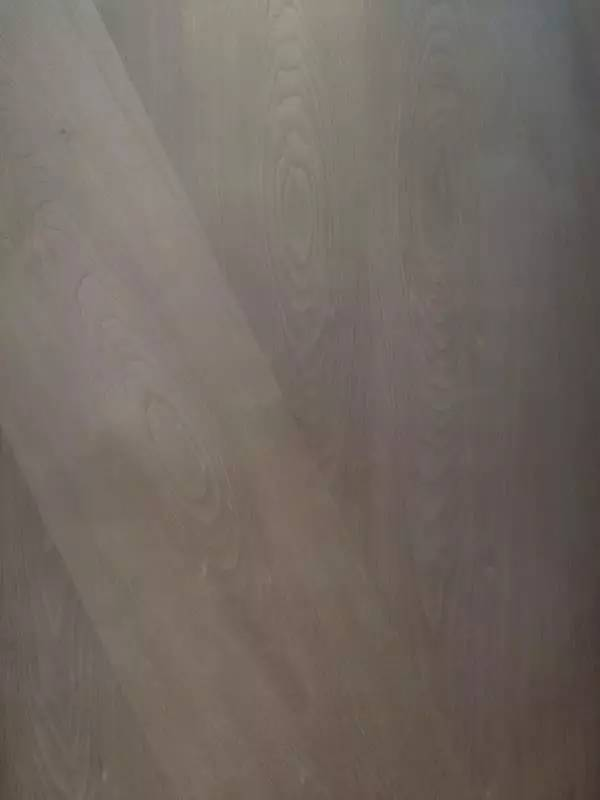精致的拼花。这是他们新做的卫浴系统，里面挤满了参观的人。设计非常特别，很好地融入了中国传统和新中式风味，很有中国水墨山水画的感觉，是全国首创。

正如木里木外产品的木饰面没有打胶和枪钉，这次设计的大理石也是挂在墙上的，很轻巧，可以随时取下来，如果不喜欢这款可以拿下来再换，增加家的多变性。简洁的收纳架是很多古装片中常见的结构，进行了现代化，采用了金属，增加了山水意蕴。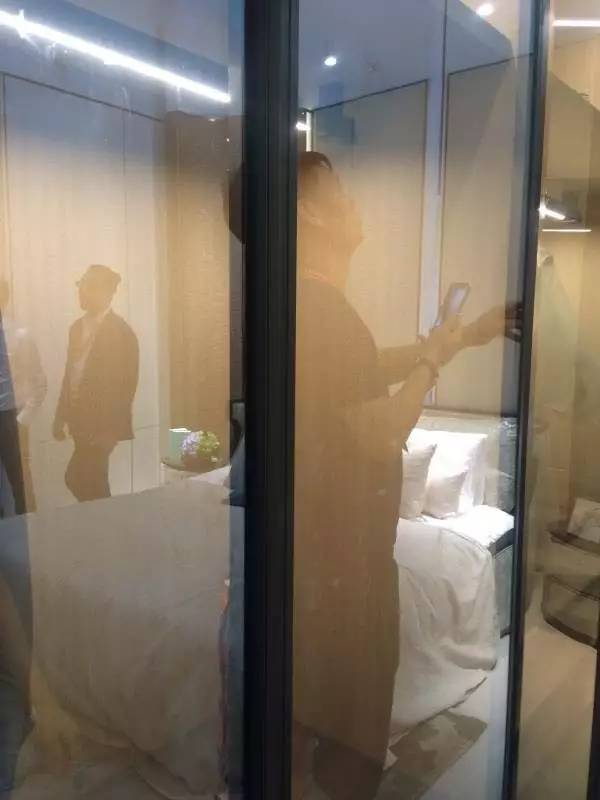木里木外的设计一直是男女衣帽间分开的，这是女衣帽间，新研发的玻璃的金属框柜门，滑轨采用世界顶端产品，叫萨迪奇，推拉非常轻松。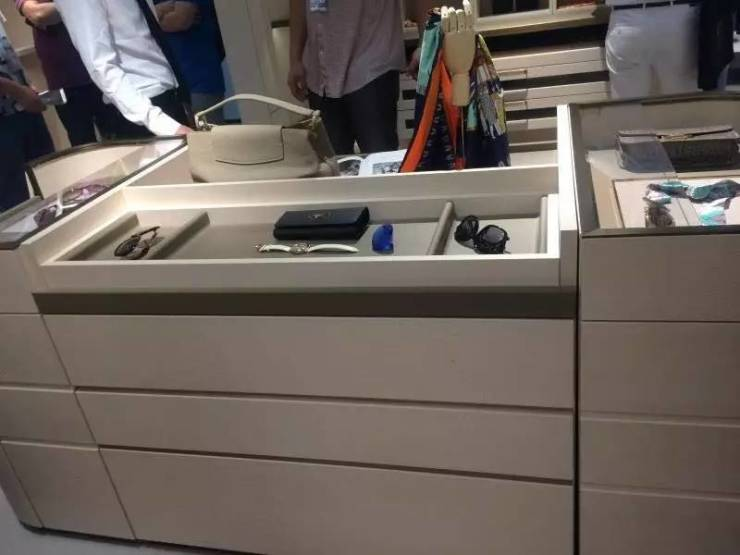洗浴间的收纳柜更加完善，满足了高端人士的各种收纳需求。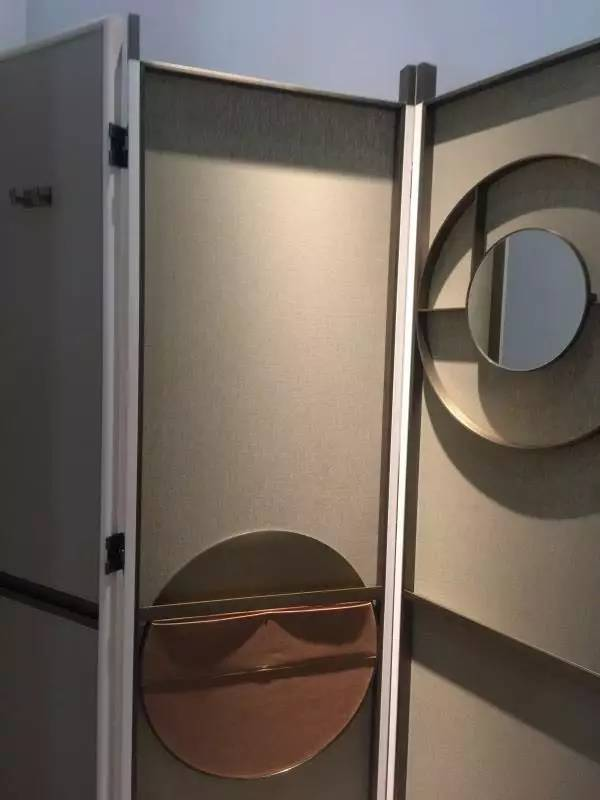这是一扇屏风，功能性很强，有镜子，可以收纳，可以挂小东西，承重能力没有问题。酒柜下增加了保温箱。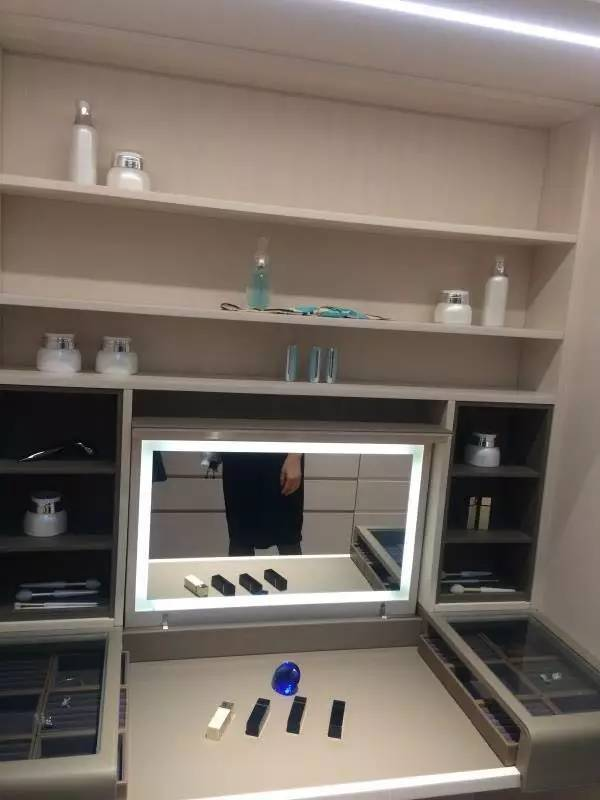新做的梳妆台，自带LED显示灯，收纳系统非常好，人性化，考虑全面。

KD定制家居：强大的收纳功能北京的KD定制家居，今年的新品更加注重收纳功能，无论是衣柜、电视柜还是衣帽间，无论大的衣物、小的首饰，都被井井有条地分配好。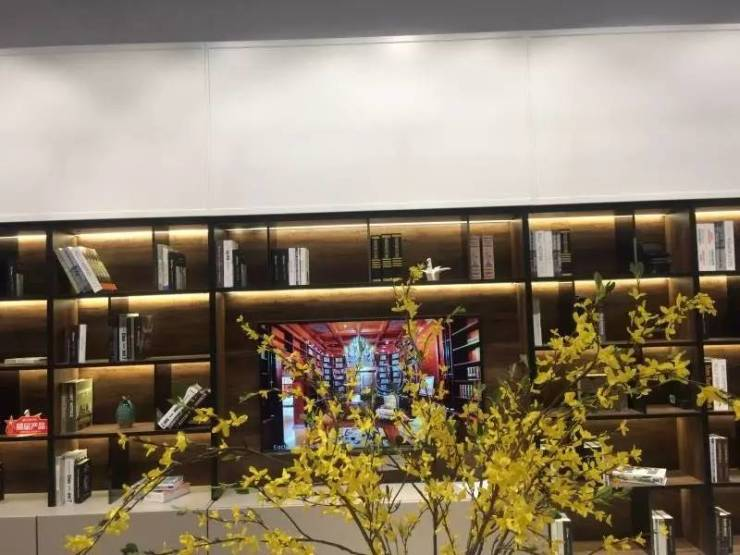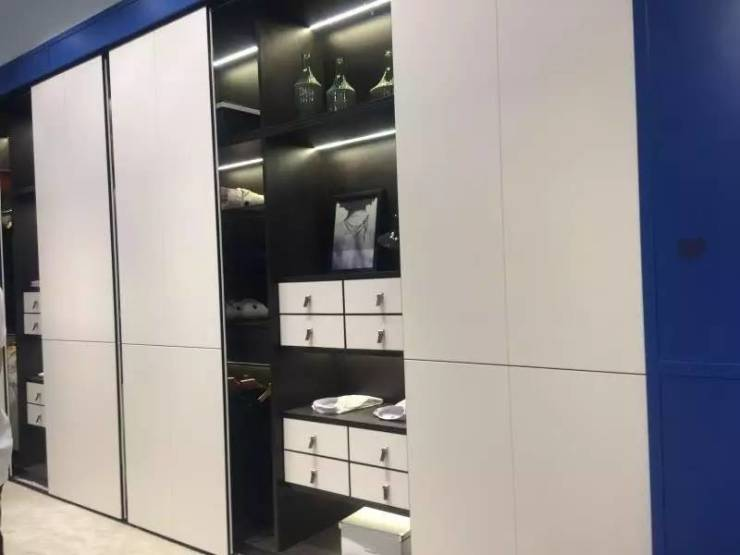现代风格的柜体设计，不仅注重设计感，更注重功能性。材质选取天然橡木和北美椴木为主，更加贴近自然。

03

材料更新颖、更环保

巴迪斯吊顶：新材料、新工艺齐登场

佛山巴迪斯新型建材有限公司在吊顶和墙壁行业的发展已经非常成熟，尤其以新中式的设计为消费者喜爱，这次展会上，他们又增加了许多新工艺和新设计。通过光氧化工艺完成的吊顶，抗辐射能力很强。这款吊顶集合了四种工艺，滚涂、加印、光氧化，边缘是水转印。这款装饰性吊顶是PU的皮质，后面是竹木纤维冲压的。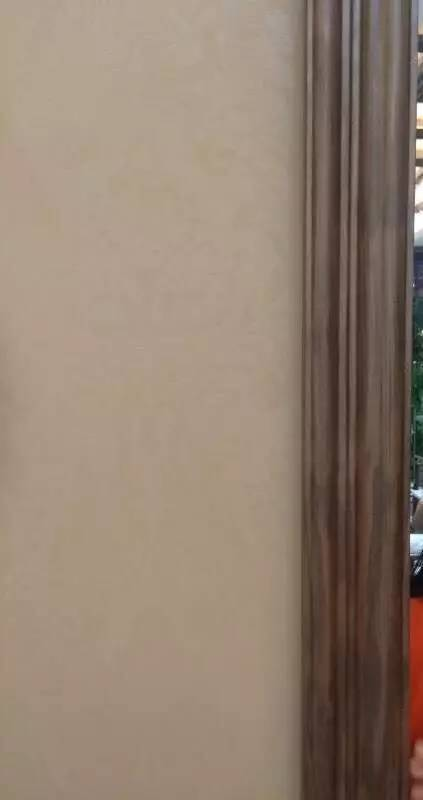这款吊顶全部采用竹木纤维表面，花纹纹理细致，镶嵌在墙壁表面，省时省力，可拆卸，施工很快。多层实木门框，质感很好。

大地硅藻泥：更加环保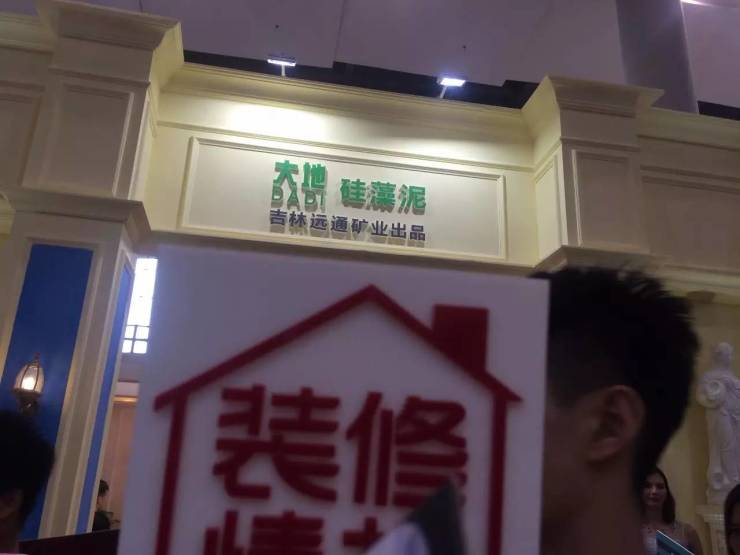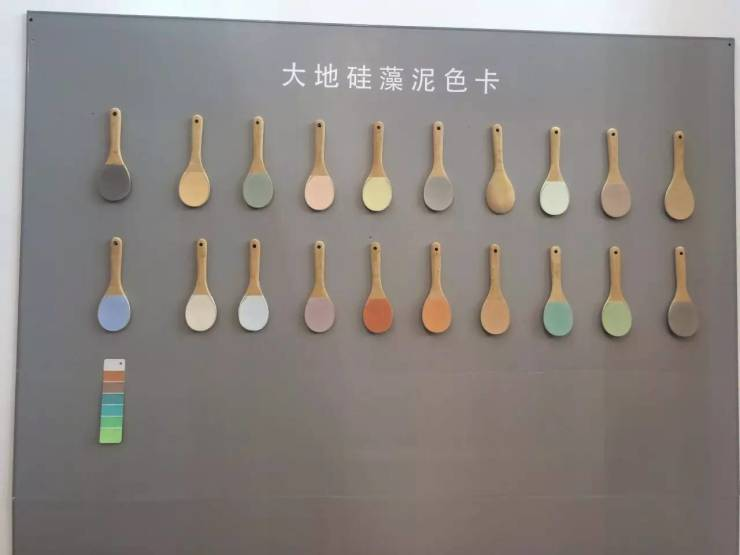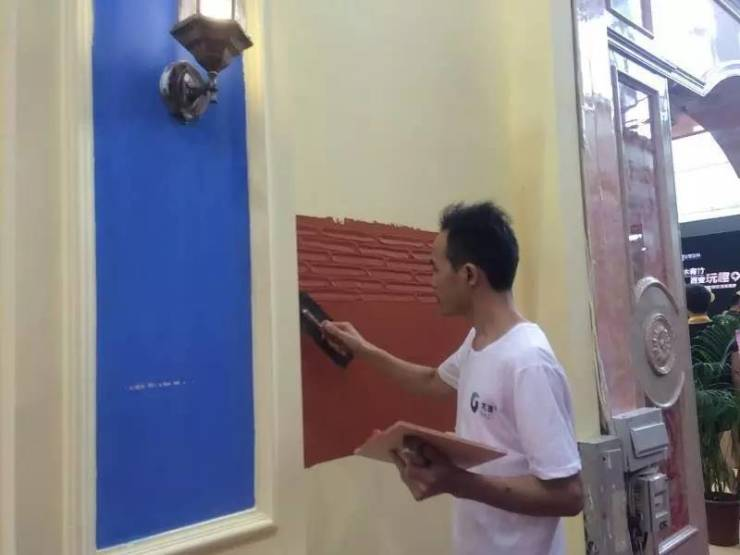大地牌硅藻泥带来了独家专利技术，添加特有的A+硅藻土，真正含30%硅藻土，在甲醛净化、防霉等方面具有非常好的性能，对幼儿园也非常试用，而且颜色多样可选。

04

满足美观需要，设计更实用

贝克洛门窗：通体铝设计是亮点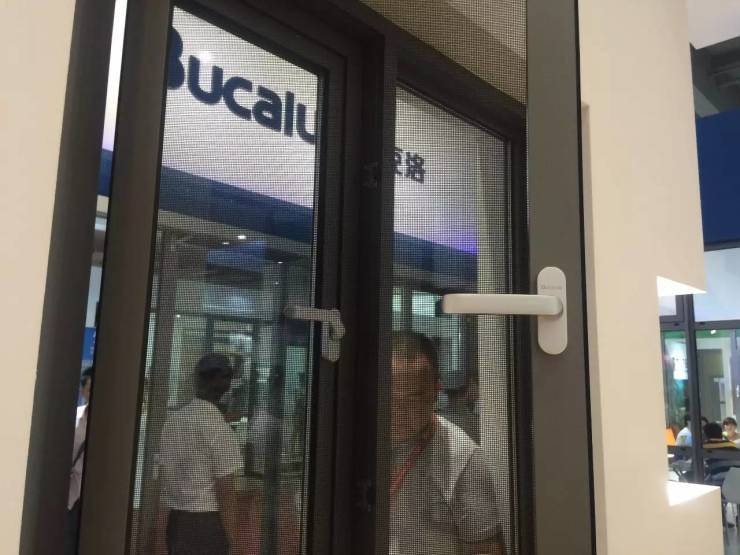通体铝设计是贝克洛门窗今年的亮点，纱窗和窗一体化，纱窗也采用铝合金，外观、设计都非常简洁，很多位于郊区的别墅非常试用，而且性价比很高。

巴迪斯吊顶：设计样式更丰富这款是拿过很多设计大奖的吊顶，更突出设计感，边框都石实木木纹。很多中式设计都会来参考。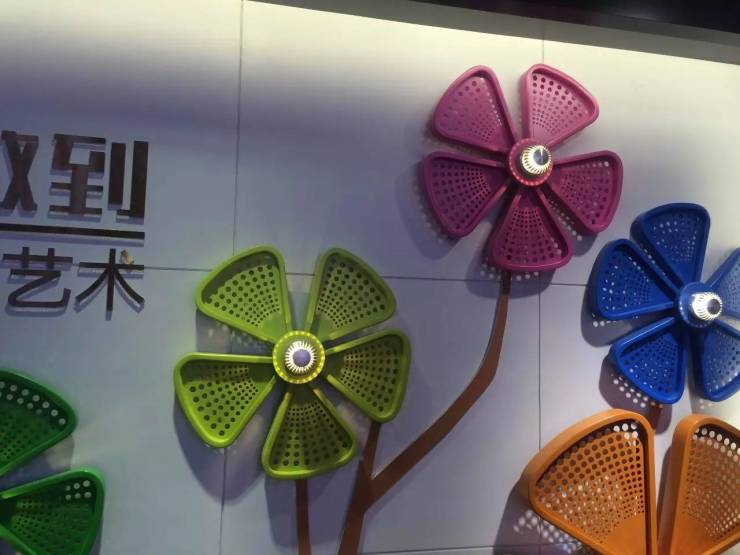展厅外面的装饰都非常讲究，墙壁的花全部是数控机床做出来的。表面采用喷涂工艺，材料是铝，比较轻巧，抗老化能力很强。

编辑留言：展会第一天，编辑将这些最有看头的新品推荐给你，紧接着，编辑还会分享更多的展会新品给大家哦，敬请期待！而对于本届展会展出的家居新品该如何更好地融入你的家，可以向编辑咨询哦。

`声明：本文由入驻焦点开放平台的作者撰写，除焦点官方账号外，观点仅代表作者本人，不代表焦点立场错误信息举报电话： 400-099-0099，邮箱：jubao@vip.sohu.com，或点此进行意见反馈，或点此进行举报投诉。`A B C D E F G H J K L M N P Q R S T W X Y Z
A - B - C - D - E
• A
• 鞍山
• 安庆
• 安阳
• 安顺
• 安康
• 澳门
• B
• 北京
• 保定
• 包头
• 巴彦淖尔
• 本溪
• 蚌埠
• 亳州
• 滨州
• 北海
• 百色
• 巴中
• 毕节
• 保山
• 宝鸡
• 白银
• 巴州
• C
• 承德
• 沧州
• 长治
• 赤峰
• 朝阳
• 长春
• 常州
• 滁州
• 池州
• 长沙
• 常德
• 郴州
• 潮州
• 崇左
• 重庆
• 成都
• 楚雄
• 昌都
• 慈溪
• 常熟
• D
• 大同
• 大连
• 丹东
• 大庆
• 东营
• 德州
• 东莞
• 德阳
• 达州
• 大理
• 德宏
• 定西
• 儋州
• 东平
• E
• 鄂尔多斯
• 鄂州
• 恩施
F - G - H - I - J
• F
• 抚顺
• 阜新
• 阜阳
• 福州
• 抚州
• 佛山
• 防城港
• G
• 赣州
• 广州
• 桂林
• 贵港
• 广元
• 广安
• 贵阳
• 固原
• H
• 邯郸
• 衡水
• 呼和浩特
• 呼伦贝尔
• 葫芦岛
• 哈尔滨
• 黑河
• 淮安
• 杭州
• 湖州
• 合肥
• 淮南
• 淮北
• 黄山
• 菏泽
• 鹤壁
• 黄石
• 黄冈
• 衡阳
• 怀化
• 惠州
• 河源
• 贺州
• 河池
• 海口
• 红河
• 汉中
• 海东
• 怀来
• I
• J
• 晋中
• 锦州
• 吉林
• 鸡西
• 佳木斯
• 嘉兴
• 金华
• 景德镇
• 九江
• 吉安
• 济南
• 济宁
• 焦作
• 荆门
• 荆州
• 江门
• 揭阳
• 金昌
• 酒泉
• 嘉峪关
K - L - M - N - P
• K
• 开封
• 昆明
• 昆山
• L
• 廊坊
• 临汾
• 辽阳
• 连云港
• 丽水
• 六安
• 龙岩
• 莱芜
• 临沂
• 聊城
• 洛阳
• 漯河
• 娄底
• 柳州
• 来宾
• 泸州
• 乐山
• 六盘水
• 丽江
• 临沧
• 拉萨
• 林芝
• 兰州
• 陇南
• M
• 牡丹江
• 马鞍山
• 茂名
• 梅州
• 绵阳
• 眉山
• N
• 南京
• 南通
• 宁波
• 南平
• 宁德
• 南昌
• 南阳
• 南宁
• 内江
• 南充
• P
• 盘锦
• 莆田
• 平顶山
• 濮阳
• 攀枝花
• 普洱
• 平凉
Q - R - S - T - W
• Q
• 秦皇岛
• 齐齐哈尔
• 衢州
• 泉州
• 青岛
• 清远
• 钦州
• 黔南
• 曲靖
• 庆阳
• R
• 日照
• 日喀则
• S
• 石家庄
• 沈阳
• 双鸭山
• 绥化
• 上海
• 苏州
• 宿迁
• 绍兴
• 宿州
• 三明
• 上饶
• 三门峡
• 商丘
• 十堰
• 随州
• 邵阳
• 韶关
• 深圳
• 汕头
• 汕尾
• 三亚
• 三沙
• 遂宁
• 山南
• 商洛
• 石嘴山
• T
• 天津
• 唐山
• 太原
• 通辽
• 铁岭
• 泰州
• 台州
• 铜陵
• 泰安
• 铜仁
• 铜川
• 天水
• 天门
• W
• 乌海
• 乌兰察布
• 无锡
• 温州
• 芜湖
• 潍坊
• 威海
• 武汉
• 梧州
• 渭南
• 武威
• 吴忠
• 乌鲁木齐
X - Y - Z
• X
• 邢台
• 徐州
• 宣城
• 厦门
• 新乡
• 许昌
• 信阳
• 襄阳
• 孝感
• 咸宁
• 湘潭
• 湘西
• 西双版纳
• 西安
• 咸阳
• 西宁
• 仙桃
• 西昌
• Y
• 运城
• 营口
• 盐城
• 扬州
• 鹰潭
• 宜春
• 烟台
• 宜昌
• 岳阳
• 益阳
• 永州
• 阳江
• 云浮
• 玉林
• 宜宾
• 雅安
• 玉溪
• 延安
• 榆林
• 银川
• Z
• 张家口
• 镇江
• 舟山
• 漳州
• 淄博
• 枣庄
• 郑州
• 周口
• 驻马店
• 株洲
• 张家界
• 珠海
• 湛江
• 肇庆
• 中山
• 自贡
• 资阳
• 遵义
• 昭通
• 张掖
• 中卫

1室1厅1厨1卫1阳台

1
2
3
4
5

0
1
2

1

1

0
1
2
3报名成功，资料已提交审核A B C D E F G H J K L M N P Q R S T W X Y Z
A - B - C - D - E
• A
• 鞍山
• 安庆
• 安阳
• 安顺
• 安康
• 澳门
• B
• 北京
• 保定
• 包头
• 巴彦淖尔
• 本溪
• 蚌埠
• 亳州
• 滨州
• 北海
• 百色
• 巴中
• 毕节
• 保山
• 宝鸡
• 白银
• 巴州
• C
• 承德
• 沧州
• 长治
• 赤峰
• 朝阳
• 长春
• 常州
• 滁州
• 池州
• 长沙
• 常德
• 郴州
• 潮州
• 崇左
• 重庆
• 成都
• 楚雄
• 昌都
• 慈溪
• 常熟
• D
• 大同
• 大连
• 丹东
• 大庆
• 东营
• 德州
• 东莞
• 德阳
• 达州
• 大理
• 德宏
• 定西
• 儋州
• 东平
• E
• 鄂尔多斯
• 鄂州
• 恩施
F - G - H - I - J
• F
• 抚顺
• 阜新
• 阜阳
• 福州
• 抚州
• 佛山
• 防城港
• G
• 赣州
• 广州
• 桂林
• 贵港
• 广元
• 广安
• 贵阳
• 固原
• H
• 邯郸
• 衡水
• 呼和浩特
• 呼伦贝尔
• 葫芦岛
• 哈尔滨
• 黑河
• 淮安
• 杭州
• 湖州
• 合肥
• 淮南
• 淮北
• 黄山
• 菏泽
• 鹤壁
• 黄石
• 黄冈
• 衡阳
• 怀化
• 惠州
• 河源
• 贺州
• 河池
• 海口
• 红河
• 汉中
• 海东
• 怀来
• I
• J
• 晋中
• 锦州
• 吉林
• 鸡西
• 佳木斯
• 嘉兴
• 金华
• 景德镇
• 九江
• 吉安
• 济南
• 济宁
• 焦作
• 荆门
• 荆州
• 江门
• 揭阳
• 金昌
• 酒泉
• 嘉峪关
K - L - M - N - P
• K
• 开封
• 昆明
• 昆山
• L
• 廊坊
• 临汾
• 辽阳
• 连云港
• 丽水
• 六安
• 龙岩
• 莱芜
• 临沂
• 聊城
• 洛阳
• 漯河
• 娄底
• 柳州
• 来宾
• 泸州
• 乐山
• 六盘水
• 丽江
• 临沧
• 拉萨
• 林芝
• 兰州
• 陇南
• M
• 牡丹江
• 马鞍山
• 茂名
• 梅州
• 绵阳
• 眉山
• N
• 南京
• 南通
• 宁波
• 南平
• 宁德
• 南昌
• 南阳
• 南宁
• 内江
• 南充
• P
• 盘锦
• 莆田
• 平顶山
• 濮阳
• 攀枝花
• 普洱
• 平凉
Q - R - S - T - W
• Q
• 秦皇岛
• 齐齐哈尔
• 衢州
• 泉州
• 青岛
• 清远
• 钦州
• 黔南
• 曲靖
• 庆阳
• R
• 日照
• 日喀则
• S
• 石家庄
• 沈阳
• 双鸭山
• 绥化
• 上海
• 苏州
• 宿迁
• 绍兴
• 宿州
• 三明
• 上饶
• 三门峡
• 商丘
• 十堰
• 随州
• 邵阳
• 韶关
• 深圳
• 汕头
• 汕尾
• 三亚
• 三沙
• 遂宁
• 山南
• 商洛
• 石嘴山
• T
• 天津
• 唐山
• 太原
• 通辽
• 铁岭
• 泰州
• 台州
• 铜陵
• 泰安
• 铜仁
• 铜川
• 天水
• 天门
• W
• 乌海
• 乌兰察布
• 无锡
• 温州
• 芜湖
• 潍坊
• 威海
• 武汉
• 梧州
• 渭南
• 武威
• 吴忠
• 乌鲁木齐
X - Y - Z
• X
• 邢台
• 徐州
• 宣城
• 厦门
• 新乡
• 许昌
• 信阳
• 襄阳
• 孝感
• 咸宁
• 湘潭
• 湘西
• 西双版纳
• 西安
• 咸阳
• 西宁
• 仙桃
• 西昌
• Y
• 运城
• 营口
• 盐城
• 扬州
• 鹰潭
• 宜春
• 烟台
• 宜昌
• 岳阳
• 益阳
• 永州
• 阳江
• 云浮
• 玉林
• 宜宾
• 雅安
• 玉溪
• 延安
• 榆林
• 银川
• Z
• 张家口
• 镇江
• 舟山
• 漳州
• 淄博
• 枣庄
• 郑州
• 周口
• 驻马店
• 株洲
• 张家界
• 珠海
• 湛江
• 肇庆
• 中山
• 自贡
• 资阳
• 遵义
• 昭通
• 张掖
• 中卫• 手机• 分享
• 设计
免费设计
• 计算器
装修计算器
• 入驻
合作入驻
• 联系
联系我们
• 置顶
返回顶部